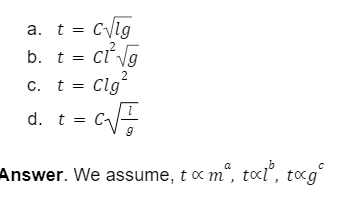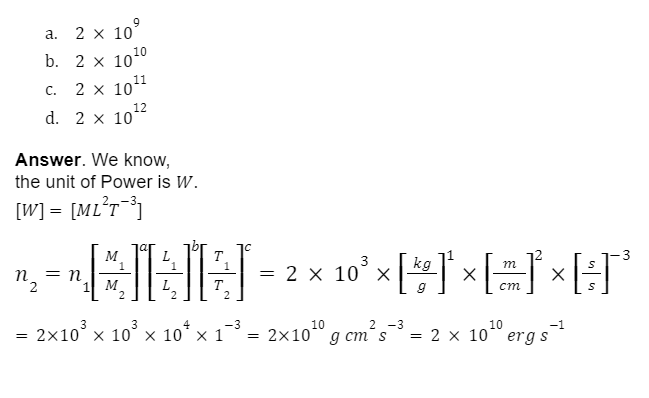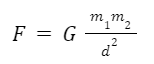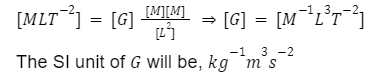• Call Now

1800-102-2727•

# Dimensional Analysis: Applications, Principle of Homogeneity, Limitations, FAQs

Have you ever wondered how the terms associated with an equation in physics relate to each other? Can the terms signify different physical quantities when they are added together or subtracted from each other? Can they be multiplied or divided? Let’s try to find answers to these questions in dimensional analysis and its applications.

Every physical quantity has a dimension which represents its constituent fundamental quantities. By dimensional analysis we can understand the significance of a physical quantity and what its unit will be. In many cases the dimensional analysis can be used to get relations between different physical quantities.

• List of Applications of Dimensional Analysis
• Checking the Correctness of the Given Physical Relation
• Principle of Homogeneity
• Conversion of System of Units
• Deriving the Relationship Between Various Physical Quantities
• Finding the Dimension and Unit of a Physical Constant
• Limitations of Dimensional Analysis
• Practice Problems of Dimensional Analysis
• FAQs of Dimensional Analysis

## List of Applications of Dimensional Analysis

• Checking the dimensional correctness of the given physical relation
• conversion of system of Units
• Deriving the relationship between various physical quantities
• Finding the dimensions of a physical constant or coefficient
• Finding the unit of a physical quantity

## Checking the Dimensional Correctness of the Given Physical Relation

For a physical relation to be correct, the dimensions of the fundamental quantities of each term on both sides of the physical relation must be the same.

## Principle of Homogeneity

• The magnitude of physical quantities may be added together or subtracted from one another only if they have the same dimensions.
• any physical quantity can be multiplied or divided with the same or different dimensions.

For an example 1 km and 2 s cannot be added or subtracted but they can be multiplied or divided.

## Conversion of System of Units

Let n1 and n2 be the numerical values of a physical quantity and u1 and u2 be their units in two different systems of units. Then,

nu = Constant

n1u1 = n2u2

n1[M1aL1bT1c] =n2[M2aL2bT2c]

## Deriving the Relationship Between Various Physical Quantities

• Establishing a relationship between different physical quantities.
• If the various factors on which a physical quantity depends are known then we can find a relation among the factors by using the principle of homogeneity.

## Finding the Dimension and Unit of a Physical Constant

In many cases the physical equation is derived from the relationships of the variables involved. In those cases a proportionality constant is taken to complete the equation. Thus we get many physical constants like Gravitational constant, Plank’s constant etc. The dimensions of such constants can be derived from the dimensions of the terms involved in that equation. From this dimension we get the unit.

## Limitations of Dimensional Analysis

Dimension does not depend on the magnitude of a physical quantity. Thus, a dimensionally correct equation need not be physically correct (by values).

The constant of proportionality of any equation cannot be deduced by the method of dimensional analysis.

This method is only applicable if the relation is of the multiplication or division type. It fails in the case of addition, subtraction, exponential and trigonometric relations.

Note: Quantities in the powers and inside the argument of trigonometric functions are dimensionless.

## Practice Problems of Dimensional Analysis

Question.1  Find the dimensions of A and B in the equation v = A + Bt, where v is the velocity and t is the time.

a. [A] = [LT-2] and [B] = LT-1

b. [A] = [LT-1] and [B] = [LT-2]

c. [A] = [L2T-1] and [B] = [LT-1]

d. [A] = [LT-1] and [B] = [L2T-2]

Answer. v = A + Bt

Using the principle of homogeneity,

Dimension of v = Dimension of A = Dimension of Bt

Dimension of A = Dimension of v

[A] = [LT-1]

Thus we can say that the term A is of velocity.

Dimension of Bt = Dimension of v

[B ][T] = [LT-1]

[B ] = [LT-2]

Thus we can say that the term B is of acceleration.

Question.2  Find the dimensional formula of X in the equation A=A0eXt , where A and A0 are the pressures and t is the time.

a. [X] = [ML2T-1]

b. [X]= [LT-1]

c. [X] = [ML2T-2]

d. [X] = [T-1

Quantities in the powers are dimensionless.

Dimension of Xt,

Xt=M0L0T0

XT=M0L0T0

X=[T-1]

Question.3 Find an expression for the time period (t) of a simple pendulum. The time period (t) may depend upon mass m of the bob of the pendulum, length l of the pendulum, and acceleration due to gravity g at the place where the bob is suspended. (Take C as the constant of proportionality.)

1.Combining all the three factors,Question.4  Power of a car is 2  kW . Express it in erg s-1. Here, erg s-1 is the CGS unit of power.

1.## FAQs of Dimensional Analysis

Question.1 What are the limitations of dimensional analysis?

• a dimensionally correct equation need not be physically correct (by values).
• The proportionality constant of any equation cannot be deduced by the method of dimensional analysis.
• It is only applicable if the relation is of the multiplication or division type.

Question.2  What is the principle of homogeneity ?

Answer. The principle of homogeneity tells us that,

• The magnitude of physical quantities may be added together or subtracted from one another only if they have the same dimensions.
• Any physical quantity can be multiplied or divided with the same or different dimensions.

As an example 1 kg and 2 m cannot be added or subtracted but they can be multiplied or divided.

Question.3  The distance travelled in n th second can be written as sn=u + 12a(2n-1). Is it dimensionally correct? Discuss.

Answer. Here the dimension of the term on the left side is of length.

The terms on the right side also mean length. Here the time term of 1 s is not written that gives rise to the confusion. Actually it is, u(1 s)   + (12) a(2n -1)(1 s) . So it is correct.

Question.4  Deduce the unit of Gravitational constant, G.

Answer. From the Newton’s law of gravitation,Where F is the gravitational force, m1 and m2 are the mass of two objects, d is the distance between their centres of gravity.

So dimensionally it will be,## NCERT Class 11 Physics Chapters